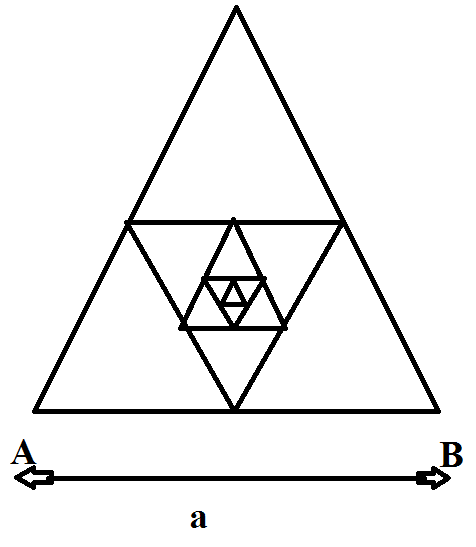# Infinite triangular ResistanceDetermine the resistance ${ R }_{ AB }$ between the points A and B of the frame made of thin homogeneous wire (as shown in figure), assuming that the number of successively embedded equilateral triangles (with sides decreasing by half) tends to infinity. Side $AB$ is equal to $a$ units and the resistance of unit length of wire is $\rho$ units.

If the resistance ${ R }_{ AB }$ is of the form $a\rho \left( \dfrac { \sqrt { d } -c }{ f } \right)$, find $d+c+f$.

×